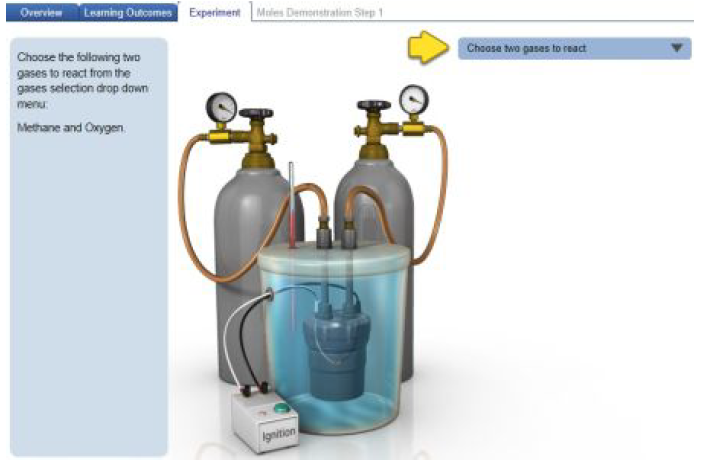# Problem: After going through a guided tutorial by selecting Run Moles Demonstration, you can create your own experiment by clicking the Run Experiment button at the end, or by clicking theOverview tab and returning to the Experiment tab to select Run Experiment. There are nine reactions you can explore on your own. Nitrogen dioxide (NO2) can be formed by reacting nitrogen monoxide gas (NO) with oxygen gas (O2). The unbalanced reaction is depicted in the diagram below. Balance the equation by placing the appropriate coefficients (including the coefficient of 1, if applicable).Drag the appropriate coefficients to their respective targets.Balanced chemical equations, which represent reactions, include coefficients that describe the ratio between the reactants and products. A balanced chemical equation not only describes how many molecules or moles react with each other, but it also embodies the fact that matter and mass are conserved in a reaction. This means that all of the atoms present among the reactants will be present in the same amount among the products. For example, the combustion reaction between methane and oxygen can be depicted in a balanced chemical equation:CH4(g) + 2O2(g) → CO2(g) + 2 H2O(g)Click on the image below to explore this simulation, which allows you run reactions between various gases and specify their amounts. When you click the simulation link, you will be able toselect among Overview, Learning Outcomes, and Experiment tabs.When the simulation is opened, you should see an overview describing how stoichiometry relates to the changes in the amounts of reactants and products in a reaction. Clicking the menu tabs toward the top of the screen can switch among an overview of stoichiometry, the learning outcomes of the simulation, and three experiments. Two of the experiments are guided tutorials that demonstrate how moles (Run Moles Demonstration) and both mass and moles (Run Grams Demonstration) change during a reaction. You can also run your own experiment by clicking Run Experiment. For any of the experiments, follow the step-by-step procedures that are explained on the left, and your interactions within the guided tutorial will be guided by an undulating yellow arrow.

###### FREE Expert Solution
100% (219 ratings)View Complete Written Solution
###### Problem Details

After going through a guided tutorial by selecting Run Moles Demonstration, you can create your own experiment by clicking the Run Experiment button at the end, or by clicking the
Overview tab and returning to the Experiment tab to select Run Experiment.

There are nine reactions you can explore on your own. Nitrogen dioxide (NO2) can be formed by reacting nitrogen monoxide gas (NO) with oxygen gas (O2). The unbalanced reaction is depicted in the diagram below. Balance the equation by placing the appropriate coefficients (including the coefficient of 1, if applicable).

Drag the appropriate coefficients to their respective targets.

Balanced chemical equations, which represent reactions, include coefficients that describe the ratio between the reactants and products. A balanced chemical equation not only describes how many molecules or moles react with each other, but it also embodies the fact that matter and mass are conserved in a reaction. This means that all of the atoms present among the reactants will be present in the same amount among the products. For example, the combustion reaction between methane and oxygen can be depicted in a balanced chemical equation:

CH4(g) + 2O2(g) → CO2(g) + 2 H2O(g)

Click on the image below to explore this simulation, which allows you run reactions between various gases and specify their amounts. When you click the simulation link, you will be able to
select among Overview, Learning Outcomes, and Experiment tabs.When the simulation is opened, you should see an overview describing how stoichiometry relates to the changes in the amounts of reactants and products in a reaction. Clicking the menu tabs toward the top of the screen can switch among an overview of stoichiometry, the learning outcomes of the simulation, and three experiments.

Two of the experiments are guided tutorials that demonstrate how moles (Run Moles Demonstration) and both mass and moles (Run Grams Demonstration) change during a reaction. You can also run your own experiment by clicking Run Experiment. For any of the experiments, follow the step-by-step procedures that are explained on the left, and your interactions within the guided tutorial will be guided by an undulating yellow arrow.